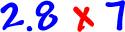There's a bit of a silly trick to multiplying decimals.  It's not really a trick -- it's just how it works -- but, it's so weird that it may seem like a trick.

It's going to sound confusing at first.  Just read through it, then look at the examples, then read it again.  It'll be really easy!

Here's the deal:

 1) Ignore the decimal points and multiply as usual... 2) Count how many total digits are on the right side of the decimal points in the guys you are multiplying... 3) Place the decimal point in your answer so there are this many digits to the right.

Let's just do one!Multiply...  Count the spots behind the decimals...  Put the decimal point in your answer:Does our answer make sense?  Do a little rounding and think about it... 2.8 is a little less than 3...  3 x 7 = 21...  So, our answer should be a little less than 21.  Yep, our answer looks good!

And remember, you can always grab a calculator to check your answers on these!